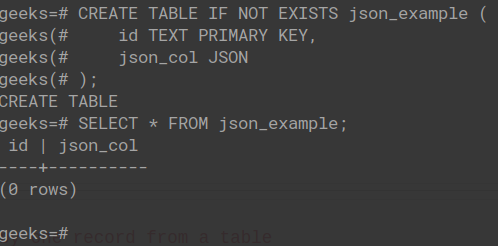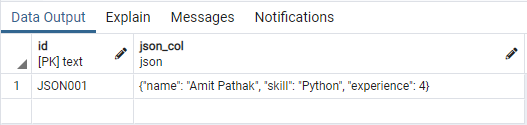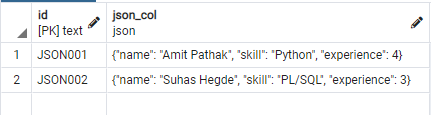GeeksforGeeks App
Open AppBrowser
Continue

# Psycopg2 – Insert dictionary as JSON

In this article, we are going to see how to insert a dictionary as JSON using Psycopg2 and Python.

Python dict objects can be or rather should be stored in database tables as JSON datatype. Since most of the SQL databases have a JSON datatype. This allows using these objects or key-value pairs in the front-end or in an API to process the request that is being placed from them respectively.

## Setting up a PostgreSQL Database and Table:

Let us create a schema in PostgreSQL and add a table to it with at least one JSON type column. We can use the following SQL script to create the same.In the above SQL script, we have created a schema name “geeks”. A table “json_example ” is created in the schema which consists of two columns, one which is the primary key has column name “id” whereas the other column has name “json_col ” which is of type JSON.

## Python

 `import` `json``import` `psycopg2`` ` `# SAVE THE DB CONFIG IN A DICT OBJECT``DATABASE_CONFIG ``=` `{``    ``"database"``: ``"geeks"``,``    ``"user"``: ``"postgres"``,``    ``"password"``: ``"password"``,``    ``"host"``: ``"localhost"``,``    ``"port"``:  ``5432``,``}`` ` ` ` `def` `get_connection():`` ` `    ``# RETURN THE CONNECTION OBJECT``    ``return` `psycopg2.connect(``        ``database``=``DATABASE_CONFIG.get(``'database'``),``        ``user``=``DATABASE_CONFIG.get(``'user'``),``        ``password``=``DATABASE_CONFIG.get(``'password'``),``        ``host``=``DATABASE_CONFIG.get(``'host'``),``        ``port``=``DATABASE_CONFIG.get(``'port'``),``    ``)`` ` ` ` `def` `dict_to_json(value: ``dict``):`` ` `    ``# CONVERT DICT TO A JSON STRING AND RETURN``    ``return` `json.dumps(value)`` ` ` ` `def` `insert_value(``id``: ``str``, json_col: ``str``, conn):`` ` `    ``# CREATE A CURSOR USING THE CONNECTION OBJECT``    ``curr ``=` `conn.cursor()`` ` `    ``# EXECUTE THE INSERT QUERY``    ``curr.execute(f``'''``        ``INSERT INTO``            ``json_table(id, json_col) ``        ``VALUES``            ``('JSON001', '{json_col}')``    ``'''``)`` ` `    ``# COMMIT THE ABOVE REQUESTS``    ``conn.commit()`` ` `    ``# CLOSE THE CONNECTION``    ``conn.close()`` ` ` ` `def` `main():`` ` `    ``# CREATE A PSYCOPG2 CONNECTION``    ``conn ``=` `get_connection()`` ` `    ``# CREATE A PYTHON DICT OBJECT FOR JSON COL``    ``dict_obj ``=` `{``        ``"name"``: ``"Amit Pathak"``,``        ``"skill"``: ``"Python"``,``        ``"experience"``: ``4``    ``}`` ` `    ``# CONVERT DICT OBJECT TO JSON STRING``    ``json_obj ``=` `dict_to_json(value``=``dict_obj)`` ` `    ``# INSERT VALUES IN THE DATABASE TABLE``    ``insert_value(``id``=``'JSON001'``, json_col``=``json_obj,``                 ``conn``=``conn)`` ` `if` `__name__ ``=``=` `'__main__'``:``    ``main()`

After running the above python file, we can head to the pgAdmin to view the following output in json_table table under public schema. You can run the following SQL script in the Query tool –

`\$ SELECT * FROM json_table;`Explanation:

The above code is written in a functional format for a better representation of the underlying steps that take place in the entire process. The get_connection() function returns the connection object to the PostgreSQL table using which we can establish a connection to the database for our operations. If the connection object cannot be established, it will return False. Now, we have a python dict object created in variable name dict_obj. We will insert this dict object into the database. But, before we insert this dict object, we need to convert the object in JSON format since the database understands JSON format and not the python dict object. Python’s in-built module json is used to convert the dict object in a JSON string format using the dumps() method. Now that we have the JSON string and the connection object, we can insert the data in the database table json_table. For this purpose, we have created a function names insert_value() which takes in 3 arguments, namely, id for the value to be inserted in id column, json_col for the value that needs to be inserted in the json_col column, and the conn parameter for providing the connection object created earlier. We can see that The insert_value() function runs the usual INSERT SQL script using the connection object. The connection is closed once the data is been inserted to the table.

## Using the psycopg2 Json adaptation

The following code demonstrates how psycopg2’s Json adaptation can be used instead of the standard json.dumps(). In order to pass a Python object to the database as a query argument, you can use the Json adapter imported from psycopg2.extras.

## Python

 `import` `psycopg2``from` `psycopg2.extras ``import` `Json`` ` ` ` `# CREATE A PSYCOPG2 CONNECTION``conn ``=` `psycopg2.connect(``*``*``{``    ``"database"` `: ``"geeks"``,``    ``"user"`     `: ``"postgres"``,``    ``"password"` `: ``"password"``,``    ``"host"`     `: ``"localhost"``,``    ``"port"`     `:  ``5432``,``})`` ` `# CREATE DICT OBJECT TO BE INSERTED TO DB``dict_obj ``=` `{``    ``"name"``: ``"Suhas Hegde"``,``    ``"skill"``: ``"PL/SQL"``,``    ``"experience"``: ``3``}`` ` `# CREATE A CURSOR USING THE CONNECTION OBJECT``curr ``=` `conn.cursor()`` ` `# EXECUTE THE INSERT QUERY``curr.execute(f``'''``    ``INSERT INTO``        ``json_table(id, json_col) ``    ``VALUES``        ``('JSON002', %s)``'''``, [Json(dict_obj)])`` ` `# COMMIT THE REQUESTS IN QUEUE``conn.commit()`` ` `# CLOSE THE CONNECTION``conn.close()`

Output: